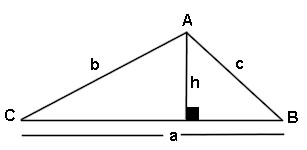# Triangle Area Side Angle Side Calculator

This calculator requires the use of Javascript enabled and capable browsers. This calculator is designed to give the area of a triangle, assuming two sides and the angle between are known, also known as SAS. Enter the two known sides and the angle. Then click on calculate. In a triangle, all interior angles total to 180 degrees. No two angles can total to 180 degrees or more. The formula is (((1/2)* a * b) * sin C). It is for any shape triangle. Click on Calculate. The unknown side, both unknown angles and the area are returned. In case you need them, here are the Trig Triangle Formula Tables, as well as the Right Triangle Angle And Side Calculator. Here is our Triangle Math Menu.

 Unit Number Required Data Entry Quantity Decimal Places Angle Units Designation degrees (decimal) radians Angle A Between Known Sides b & c Angle Units Known Side b Length Units Known Side c Length Units Calculated Results Calculated Angle B Angle Units Calculated Angle C Angle Units Calculated Side a Length Units Area Of Triangle Square UnitsUpdated 8.13.11

 Leave us a question or comment on FacebookSearch or Browse Our Site